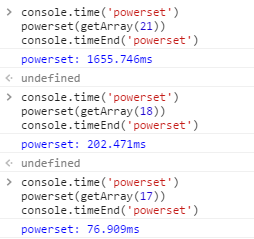# sku 多维属性状态判断算法

2016/12/22首页
§

## ¶问题描述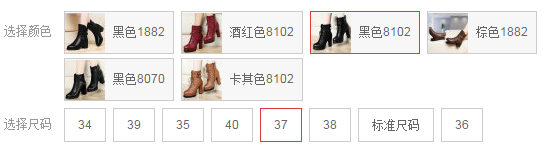``````[
{ "颜色": "红", "尺码": "大", "型号": "A", "skuId": "3158054" },
{ "颜色": "白", "尺码": "中", "型号": "B", "skuId": "3133859" },
{ "颜色": "蓝", "尺码": "小", "型号": "C", "skuId": "3516833" }
]
``````

``````{
"颜色": ["红", "白", "蓝"],
"尺码": ["大", "中", "小"],
"型号": ["A", "B", "C"]
}
``````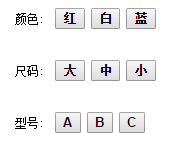``````[
["红", "大", "A"],    // ✔
["红", "大", "B"],
["红", "大", "C"],
["红", "中", "A"],
["红", "中", "B"],
["红", "中", "C"],
["红", "小", "A"],
["红", "小", "B"],
["红", "小", "C"],
["白", "大", "A"],
["白", "大", "B"],
["白", "大", "C"],
["白", "中", "A"],
["白", "中", "B"],    // ✔
["白", "中", "C"],
["白", "小", "A"],
["白", "小", "B"],
["白", "小", "C"],
["蓝", "大", "A"],
["蓝", "大", "B"],
["蓝", "大", "C"],
["蓝", "中", "A"],
["蓝", "中", "B"],
["蓝", "中", "C"],
["蓝", "小", "A"],
["蓝", "小", "B"],
["蓝", "小", "C"]     // ✔
]
``````

## ¶确定规则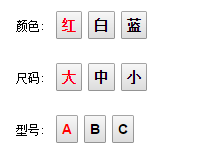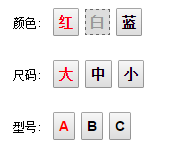...

## ¶解决方法

1. 遍历所有非已选元素：`"白", "蓝", "中", "小", "B", "C"`
1. 遍历所有属性行： `"颜色", "尺码", "型号"`
1. 取： a) 当前元素 b) 非当前元素所在的其它属性已选元素，形成一个路径
2. 判断此路径是否存在，如果不存在将当前元素置灰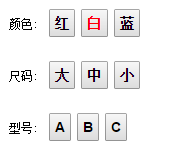### ¶缩小问题规模

• 白 - 大 - A
• 白 - 大 - B
• 白 - 大 - C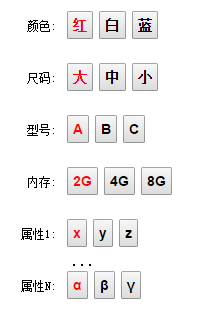### ¶调整思路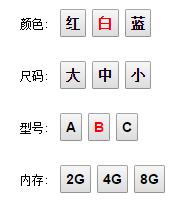图1

``````[
{ "颜色": "红", "尺码": "大", "型号": "A", "skuId": "3158054" },
{ "颜色": "白", "尺码": "中", "型号": "B", "skuId": "3133859" },
{ "颜色": "蓝", "尺码": "小", "型号": "C", "skuId": "3516833" }
]
// 即
[
[ "红", "大", "A" ],   // 存在
[ "白", "中", "B" ],   // 存在
[ "蓝", "小", "C" ]    // 存在
]
``````

• A
• 红 - 大
• 红 - A
• 大 - A
• 红 - 大 - A

• B
• 白 - 中
• 白 - B
• 中 - B
• 白 - 中 - B

...

``````/**
* 取得集合的所有子集「幂集」
arr = [1,2,3]

i = 0, ps = [[]]:
j = 0; j < ps.length => j < 1:
i=0, j=0 ps.push(ps.concat(arr)) => ps.push([].concat(1)) => 
ps = [[], ]

i = 1, ps = [[], ] :
j = 0; j < ps.length => j < 2
i=1, j=0 ps.push(ps.concat(arr)) => ps.push([].concat(2))  => 
i=1, j=1 ps.push(ps.concat(arr)) => ps.push(.concat(2)) => [1,2]
ps = [[], , , [1,2]]

i = 2, ps = [[], , , [1,2]]
j = 0; j < ps.length => j < 4
i=2, j=0 ps.push(ps.concat(arr)) => ps.push()    => 
i=2, j=1 ps.push(ps.concat(arr)) => ps.push([1, 3]) => [1, 3]
i=2, j=2 ps.push(ps.concat(arr)) => ps.push([2, 3]) => [2, 3]
i=2, j=3 ps.push(ps.concat(arr)) => ps.push([2, 3]) => [1, 2, 3]
ps = [[], , , [1,2], , [1, 3], [2, 3], [1, 2, 3]]
*/
function powerset(arr) {
var ps = [[]];
for (var i=0; i < arr.length; i++) {
for (var j = 0, len = ps.length; j < len; j++) {
ps.push(ps[j].concat(arr[i]));
}
}
return ps;
}
``````图1

• 如何确定 `红` 可选？ 只需要确定 `红-B` 可选
• 如何确定 `中` 可选？ 需要确定 `白-中-B` 可选
• 如何确定 `2G` 可选？ 需要确定 `白-B-2G` 可选

1. 遍历所有非已选元素
1. 遍历所有属性行
1. 取： a) 当前元素 b) 非当前元素所在的其它属性已选元素（如果当前属性中没已选元素，则跳过），形成一个路径
2. 判断此路径是否存在（在所有存在的路径表中查询），如果不存在将当前元素置灰

``````{
"": {
"skus": ["3158054", "3133859", "3516833"]
},
"红": {
"skus": ["3158054"]
},
"大": {
"skus": ["3158054"]
},
"红-大": {
"skus": ["3158054"]
},
"A": {
"skus": ["3158054"]
},
"红-A": {
"skus": ["3158054"]
},
"大-A": {
"skus": ["3158054"]
},
"红-大-A": {
"skus": ["3158054"]
},
"白": {
"skus": ["3133859"]
},
"中": {
"skus": ["3133859"]
},
"白-中": {
"skus": ["3133859"]
},
"B": {
"skus": ["3133859"]
},
"白-B": {
"skus": ["3133859"]
},
"中-B": {
"skus": ["3133859"]
},
"白-中-B": {
"skus": ["3133859"]
},
"蓝": {
"skus": ["3516833"]
},
"小": {
"skus": ["3516833"]
},
"蓝-小": {
"skus": ["3516833"]
},
"C": {
"skus": ["3516833"]
},
"蓝-C": {
"skus": ["3516833"]
},
"小-C": {
"skus": ["3516833"]
},
"蓝-小-C": {
"skus": ["3516833"]
}
}
``````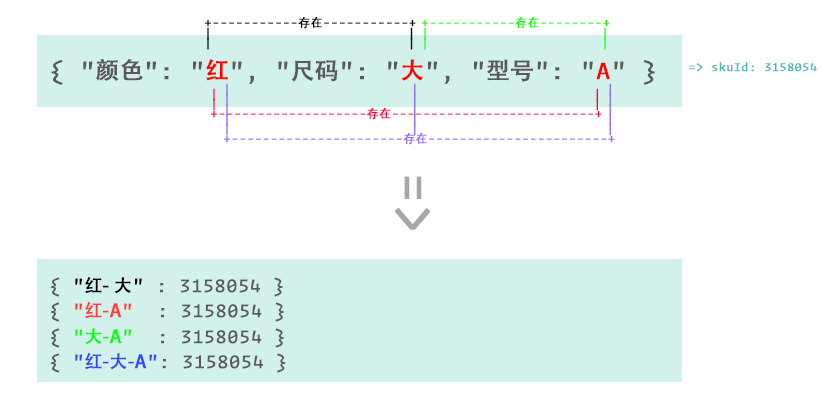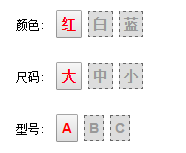### ¶优化体验

1. 无论当前属性存不存在，先高亮（选中）当前属性
2. 清除其它所有已选属性
3. 更新当前状态（只选当前属性）下的其它属性可选状态
4. 遍历非当前属性行的其它属性查找对应的在缓存中的已选属性
5. 如果缓存中对应的属性存在（可选），则默认选中缓存属性并 再次更新 其它可选状态。不存在，则高亮当前属性行（深色背景）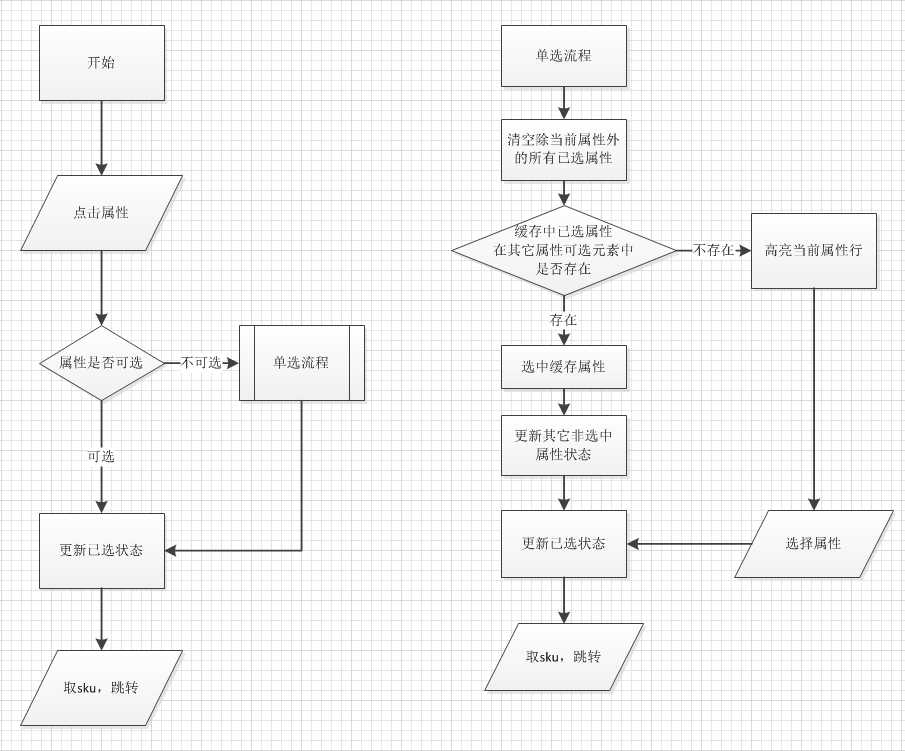``````[
{ "颜色": "红", "尺码": "大", "型号": "A", "skuId": "3158054" },
{ "颜色": "白", "尺码": "大", "型号": "A", "skuId": "3158054" }, // 多加了一条
{ "颜色": "白", "尺码": "中", "型号": "B", "skuId": "3133859" },
{ "颜色": "蓝", "尺码": "小", "型号": "C", "skuId": "3516833" }
]
``````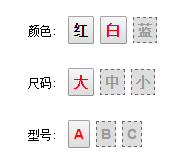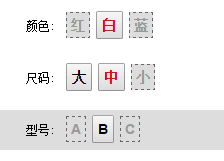## ¶算法复杂度

``````{1}       2^1 = 2
=> {},{1}
{1,2}     2^2 = 4
=> {},{1},{2},{1,2}
{1,2,3}   2^3 = 8
=> {},{1},{2},{3},{1,2},{1,3},{2,3},{1,2,3}
...
``````